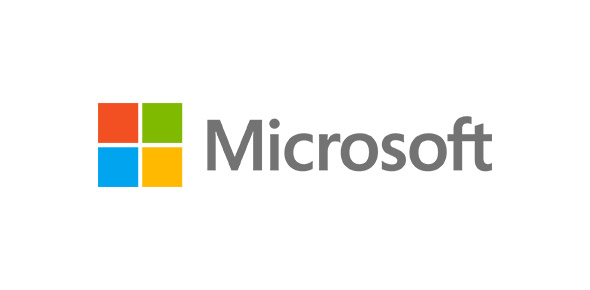# MSBI 2008 Evaluation Test

40 Questions | Attempts: 1079
ShareSettingsMSBI 2008 Evaluation Test For Cyber Think Consultants

• 1.
1.       What is the difference between UNION and UNION ALL?
• A.

A. Union selects only unique row (data) from all queries and Union all selects all rows from all queries.

• B.

B. Union selects row (data) from all queries and Union all selects all rows from all queries.

• C.

C. Union selects only unique row (data) from all queries and Union all selects rows from all queries.

• D.

D. Union selects row (data) from all queries and Union all selects rows from all queries.

• 2.
1.       Define Row Number function?
• A.

To generate sequence number.

• B.

To generate sequence number based on order by column.

• C.

To generate number.

• D.

None.

• 3.
1.       What is Normalization? Types of normalization?
• A.

Join the tables

• B.

Move the data into tables.

• C.

Split the table to reduce duplicates and it has five types.

• D.

None

• 4.
1.       What are different types of joins?
• A.

Inner, outer, self join.

• B.

Equi join and cross join.

• C.

Right outer join.

• D.

All above.

• 5.
1.       What are the advantages of using stored procedure?
• A.

A. Pre executable program.

• B.

B. We can get the data.

• C.

C. Security.

• D.

D. It is similar to function.

• 6.
1.       What is BCP?
• A.

A. Bulk copy program.

• B.

• C.

C. A and B.

• D.

D. None.

• 7.
1.       Define Index?
• A.

A. Predefined pointers to data page.

• B.

B. It is primary key.

• C.

C. It is foreign key.

• D.

D. None.

• 8.
1.       What command do we use to rename db, table, and column?
• A.

A. Invoke command

• B.

B. Commit

• C.

C. Rename

• D.

D. None.

• 9.
1.       Define having clause?
• A.

A. It is similar to where but must use group by clause.

• B.

B. It is equal to where clause.

• C.

C. None.

• D.

D. It is equal to from clause.

• 10.
1.       What are the basic functions system databases?
• A.

A. To create functions.

• B.

B. To create stored procedure.

• C.

• D.

D. None.

• 11.
1.       Explain ETL process?
• A.

• B.

• C.

C. Both a and b.

• D.

D. None.

• 12.
1.       Difference between for loop and for each loop container?
• A.

A. For loop based on conditions and for each loop based on objects collections.

• B.

B. For loop based on objects collections and for each loop based on objects collections.

• C.

C. None.

• D.

D. Both a and b.

• 13.
1.       What does a control flow do?
• A.

A. To control workflow.

• B.

B. To control events.

• C.

C. To control data flows.

• D.

D. To control lookup.

• 14.
1.       Explain event handlers?
• A.

A. To handle data validations.

• B.

B. To handle errors.

• C.

C. To handle events.

• D.

D. None.

• 15.
1.       SSIS 2008 configuration types?
• A.

A. Five.

• B.

B. Four.

• C.

C. Three.

• D.

D. One.

• 16.
1.       Define Lookup transformation?
• A.

A. Used as reference table.

• B.

B. Used as master table.

• C.

C. Used as fact table

• D.

D. None.

• 17.
1.       Define synchronous transformations?
• A.

A. Input rows count and Output rows count are same.

• B.

B. Input rows count and Output rows count are not same.

• C.

C. None.

• D.

D. Input rows count and Output rows count are different.

• 18.
1.       How to rename file using SSIS tasks?
• A.

A. Using lookup.

• B.

B. Using Data flow.

• C.

• D.

D. None.

• 19.
1.       What does mean by data auditing?
• A.

A. To maintain data load statistics in ETL process.

• B.

B. To know the errors.

• C.

C. To know the Primary keys.

• D.

D. None.

• 20.
1.       How many container tasks available in SSIS?
• A.

A. One.

• B.

B. Two.

• C.

C. Three.

• D.

D. Four.

• 21.
1.       Define role play dimensions?
• A.

A. One dimension used multiple times in the cube.

• B.

B. One dimension used in different cubes.

• C.

C. Shared across cubes.

• D.

D. None.

• 22.
1.       Define dimension usage in SSAS Cube?
• A.

A. To maintain cube data size.

• B.

B. To know the relationships between dimensions and fact.

• C.

• D.

D. None.

• 23.
1.       MDX stands?
• A.

A. Memory, Distribution, extensions.

• B.

B. Memory, dimension, expressions.

• C.

C. Multi, dimension, expressions.

• D.

D. None

• 24.
1.       DSV stands?
• A.

A. Data source View.

• B.

B. It is view.

• C.

C. It has relationships and metadata.

• D.

D. All above.

• 25.
1.       How many types of actions available in the Cube?
• A.

A. One.

• B.

B. Two.

• C.

C. Three.

• D.

D. None.

## Related TopicsBack to top
×

Wait!
Here's an interesting quiz for you.